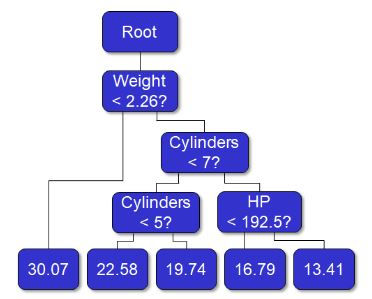## IFPOSTS

An Overview of Machine Learning Methods

Russ, W. J. and Ennis, J. M. (2018).

Machine learning is more than just a flashy buzzword - it’s a collection of powerful tools that can lead to valuable insights. So what exactly is it? Machine learning is any process whereby software programs, when given more data, improve their ability to perform tasks. Despite the science fiction sounding nature of that definition, most people have already used machine learning in some form. To illustrate this point, we begin our exposition of machine learning with some common examples.

Perhaps the simplest algorithm for machine learning is linear regression. While the term “machine learning” has only been around since 1959 (Samuel, 1959), linear regression is included even though its first uses date back to the 1800’s (Galton, 1886). Regression is a powerful tool due to its simplicity, but it can be limited by its assumptions that predictive relationships are additive (or can be transformed to be additive) and that predictor variables are not too highly correlated. More recent advancements in linear regression, such as LASSO and ridge regression, have led to automatic variable selection and handling of multicollinearity (Friedman, 2001, Ch 3.). Regression would be a good choice for modeling the relationship between number of rooms, area, age, and home value. We expect those relationships to be continuous and monotonic.

Other forms of machine learning generalize regression by dividing the space of predictor values into regions. One such example is decision trees.An example of a decision tree for engine displacement

Decision tree algorithms essentially create a flowchart to guides the model predictions. These flowcharts are often easy to understand by humans and can handle highly correlated predictor variables, but they can also be inaccurate and unstable - when the data change even a little, trees can change drastically. Random forests were created to address these stability issues. Random forest methods create many trees, each from a subset of the predictor variables, and combine their results to create a final prediction which is more stable and usually more accurate - but at the expense of interpretability (Kuhn, 2013, Ch. 8 and 14). Of interest to consumer scientists is the fact that decision trees are excellent for modeling consumer segmentation, allowing us to see which factors are most differentiating and where those factors induce splits between segments.

Another method which works by dividing the predictor space is support vector machines (SVMs). SVMs are an extension of linear discriminant analysis, and work by finding planes in the space which best divide the data into categories (James, 2013, Ch. 9). An improvement over regression-based approaches, SVM algorithms automatically transform the space of predictor values to allow for non-linear relationships. Moreover, SVMs can be used for continuous or categorical predictions, although they work best for categorical outcomes. While SVMs can be powerful for prediction, models produced by SVMs are “black box” and nearly impossible to interpret. Hence they can propagate latent bias in the training data without such bias being conspicuous. Such concerns notwithstanding, support vector machines are a good choice when you are unsure of the relationship between predictors and outcome, especially if you have a large number of predictors.

One final example of machine learning algorithms are those built on networks. Inspired by neurobiology, artificial neural networks (ANNs), which include deep learning and convolutional neural networks (CNNs), employ a network of nodes where each node implements a rule about how it transforms input into output. The outputs of individual nodes are input to other nodes until a final output gives the prediction (Géron, 2017, Ch. 10). These techniques work best when there is a large amount of data, but recent advances such as adversarial neural networks provide alternative methods of model training. Like support vector machines, ANNs are difficult to interpret but can make predictions from problems that are traditionally difficult for computers to solve, such as classifying images, playing “go” at superhuman levels, or even creating novel portraiture.

There are many other methods for machine learning, each with their strengths and weaknesses. Choosing the right method depends on the data and the type of model being constructed. Key aspects to consider are the desired transparency of the model, the relationships within the input data, the nature of the response variable, and the trade-off between variability and bias. By carefully choosing the correct one for the job, however, useful models can be built and valuable insights may be gleaned.

Interested in machine learning and its applications to consumer research?
Check out our webinar, A Three-Step Approach to Characterizing Consumer Segmentation via Machine Learning!References:

Galton, Francis. (1886). Regression Towards Mediocrity in Hereditary Stature. Journal of the Anthropological Institute, 15:246-263.

Friedman, J., Hastie, T., & Tibshirani, R. (2001). The elements of statistical learning (Vol. 1, No. 10). New York, NY, USA:: Springer series in statistics.

Géron, A. (2017). Hands-on machine learning with Scikit-Learn and TensorFlow: concepts, tools, and techniques to build intelligent systems. " O'Reilly Media, Inc.".

James, G., Witten, D., Hastie, T., & Tibshirani, R. (2013). An introduction to statistical learning (Vol. 112). New York: Springer.

Kuhn, M., & Johnson, K. (2013). Applied predictive modeling(Vol. 26). New York: Springer.

Samuel, Arthur. (1959). Some Studies in Machine Learning Using the Game of Checkers. IBM Journal of Research and Development. 3 (3): 210–229.